# Solutions of a Linear Equation

Solutions of a Linear Equation

Linear equations involve unknown quantities in the form of one or more variables to represent real-life problems.
It helps in finding out the cost, mileage, speed, and distance, etc. with ease.
We all use linear equations in our daily life without knowing.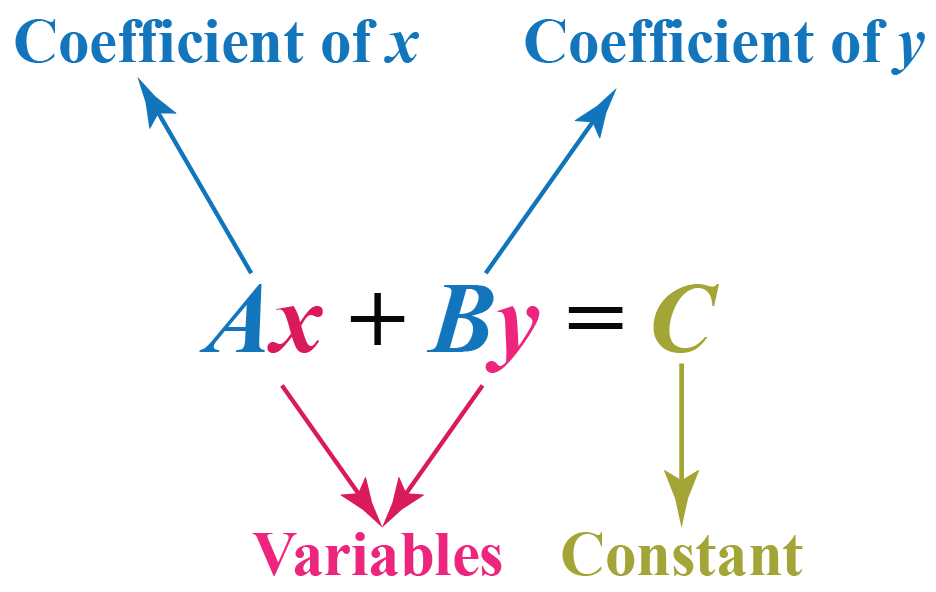Come, let us learn in detail about the solutions of linear equations, consistent and inconsistent equations, and homogenous linear equations, etc. in this mini-lesson.

## Lesson Plan

 1 What Are the Solutions of a Linear Equation? 2 Tips and Tricks 3 Solved Examples on Linear Equation 4 Challenging Questions on Linear Equation 5 Interactive Questions on Linear Equation

## What Are the Solutions of a Linear Equation?

The solutions of linear equations are the points at which the lines or planes representing the linear equations intersect or meet each other.

A solution set of a system of linear equations is the set of values to the variables of all possible solutions.

For example, while solving linear equations one can visualize the solution of a system of simultaneous linear equations by drawing 2 linear graphs and finding out their intersection point.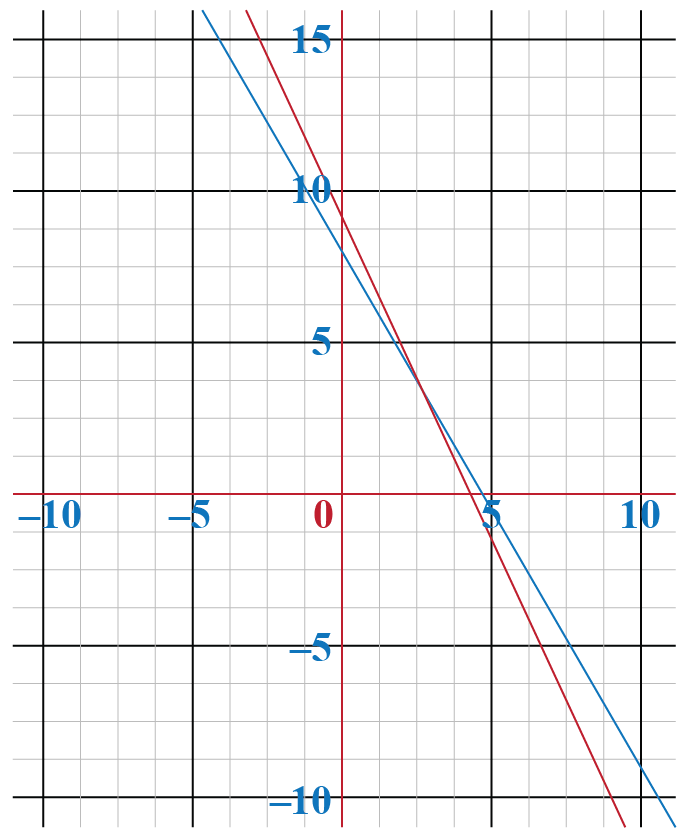The red line represents all the solutions for equation 1, and the blue line, solutions for equation 2. The intersection at a unique point (2,4) is the solution that will satisfy both equations.

## What Are the Types of Solutions for Linear Equations?

A system of linear equations can have 3 types of solutions.

### 1. Unique Solution of a system of linear equations

The unique solution of a linear equation means that there exists only one point, on substituting which, L.H.S and R.H.S of an equation become equal.
The linear equation in one variable has always a unique solution.
For example, $$3m$$=$$6$$ has a unique solution $$m$$=2 for which L.H.S = R.H.S.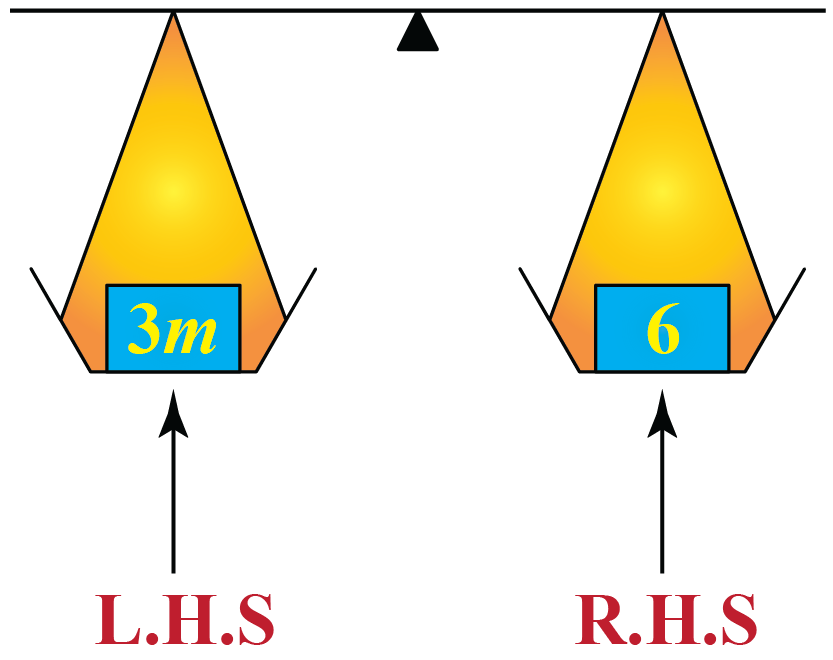Similarly, for simultaneous linear equations in two variables, the unique solution is an ordered pair (x,y) which will satisfy both the equations.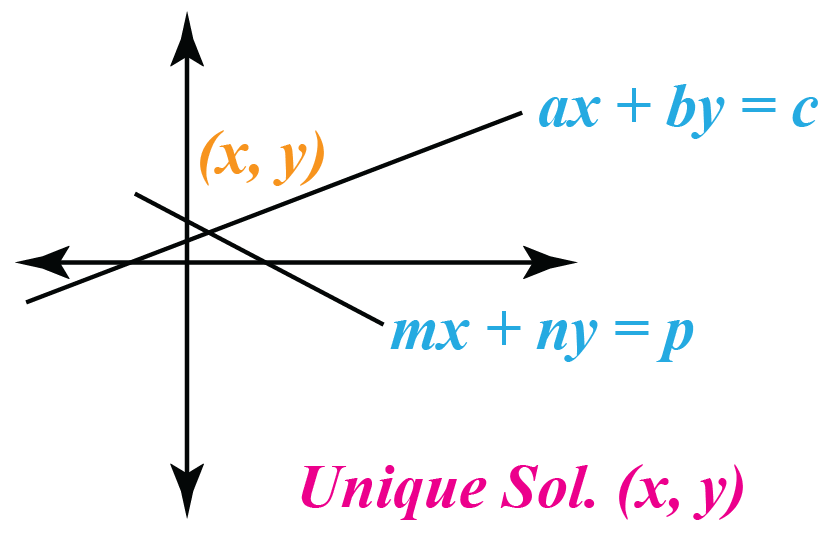### 2. No Solution

A system of linear equations has no solution when there exists no point where lines intersect each other or the graphs of linear equations are parallel.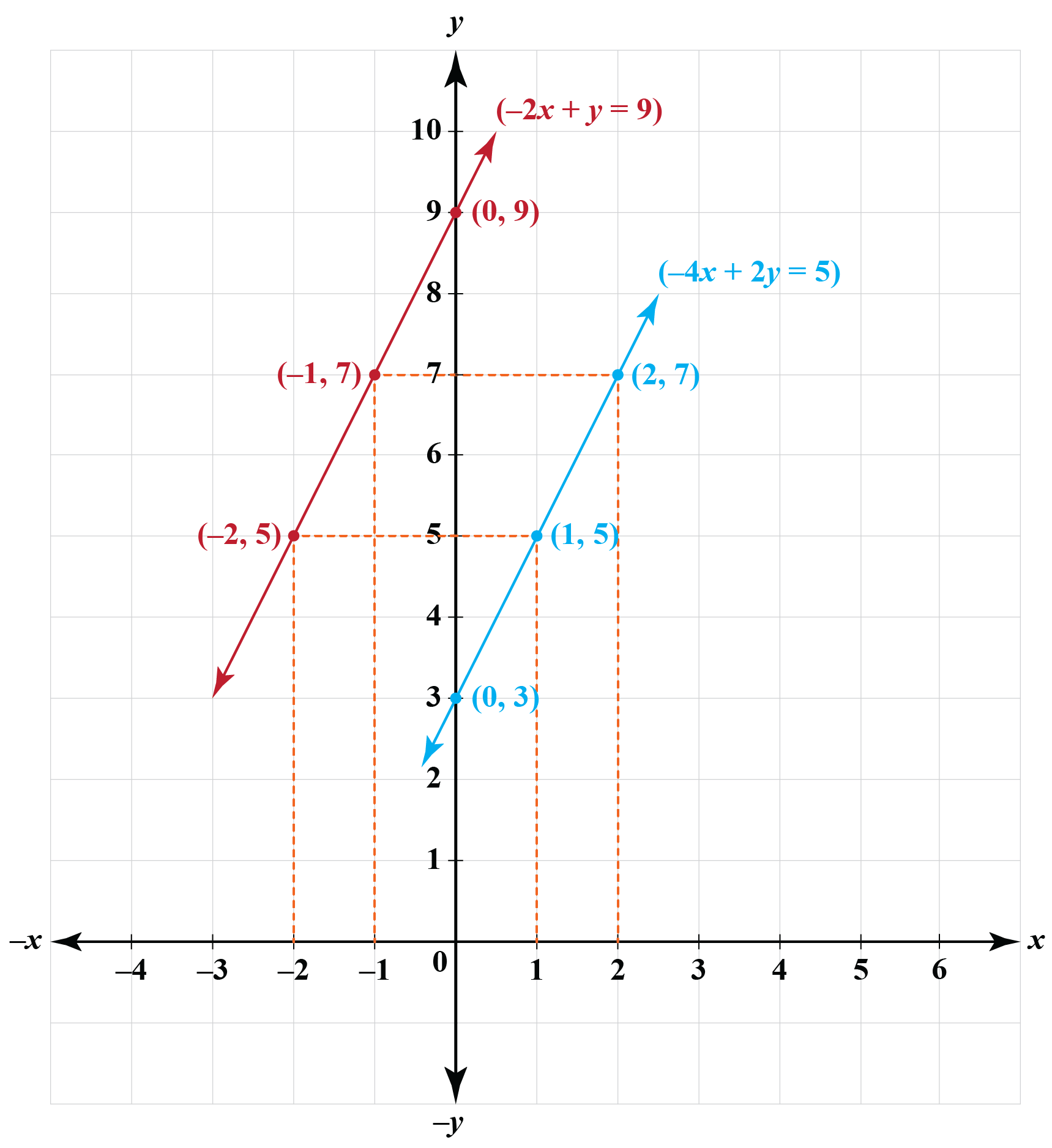### 3. Infinite Many Solutions

A system of linear equations has infinitely many solutions when there exists a solution set of infinite points for which L.H.S and R.H.S of an equation become equal, or in the graph straight lines overlap each other.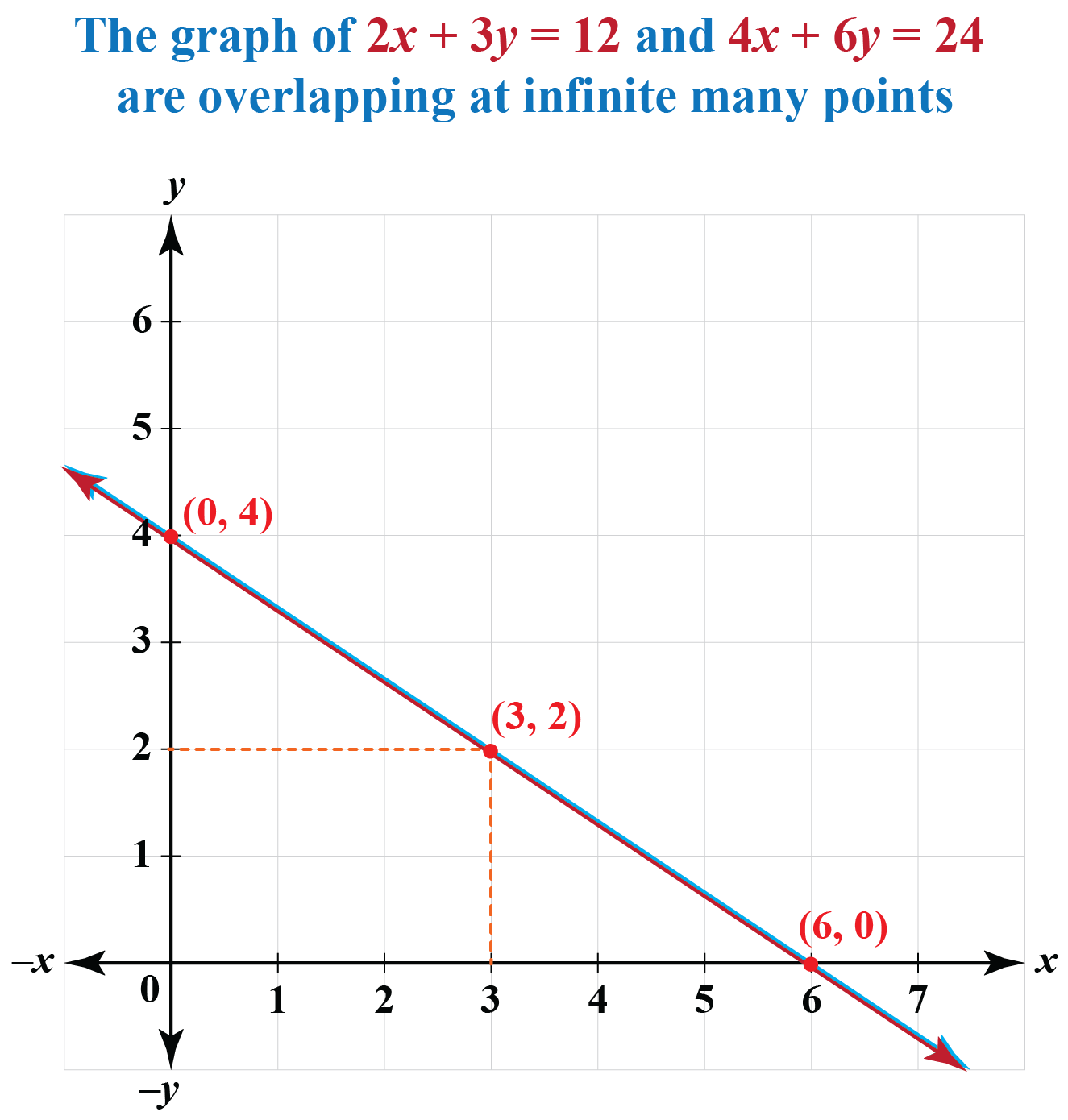## How to Find the Solution of a Linear Equation?

### Solutions for Linear Equations in One Variable

Consider the equation, $2x + 4 = 8$
To find the value of $$x$$, first, we remove $$4$$ from L.H.S, so we subtract $$4$$ from both sides of the equation.
$2x + 4 - 4 = 8 - 4$

Now we get, $2x = 4$
Now we have to remove 2 from L.H.S in order to get $$x$$, therefore we divide the equation by $$2$$
$\frac{2x}{2} = \frac{4}{2}$
$x=2$
Hence, the solution of the equation $$2x + 4 = 8$$ is $$x=2$$

### Solutions for Linear Equations in Two Variables

The following methods can be used to find the solutions of linear equations of two variables.

### 1. Substitution Method

Consider the following pair of linear equations, lets solve the following linear equation.

$\begin{array}{l}x + y = 4\;\;\;\; & ...(1)\\ x - y = 2\;\;\;\; & ...(2)\end{array}$Let’s rearrange the first equation to express y in terms of x, as follows:$\begin{array}{l}x + y = 4\\ \Rightarrow \;\;\;y = 4 - x\end{array}$

This expression for y can now be substituted in the second equation, so that we will be left with an equation in x alone:

\begin{align}& x - y = 2\\ &\Rightarrow \;\;\;x - 4 + x = 2\\ &\Rightarrow \;\;\;2x = 6\\ &\Rightarrow \;\;\;x = \frac{6}{2}\\ &\Rightarrow \;\;\;x = 3\end{align}

Once we have the value of x, we can plug this back into any of the two equations to find out y. Lets plug it into the first equation:

$\begin{array}{l}x + y = 4\\ \Rightarrow \;\;\;(3) + y = 4\\ \Rightarrow \;\;\;y = 4 - 3 = 1\\ \Rightarrow \;\;\;y = 1\end{array}$

The final non trivial solution is:

$x = 3,\;y = 1$

It should be clear why this process is called substitution. We express one variable in terms of another using one of the pair of equations, and substitute that expression into the second equation.

### 2. Elimination Method

Consider the following pair of linear equations:

$\begin{array}{l} 2x + 3y - 11 = 0\\ 3x + 2y - 9 = 0 \end{array}$

The coefficients of x in the two equations are 2 and 3 respectively. Let us multiply the first equation by 3 and the second equation by 2, so that the coefficients of x in the two equations become equal:

$\begin{array}{l}\left\{ \begin{array}{l}3 \times \left( {2x + 3y - 11 = 0} \right)\\2 \times \left( {3x + 2y - 9 = 0} \right)\end{array} \right.\\ \Rightarrow \;\;\;6x + 9y - 33 = 0\\\qquad6x + 4y - 18 = 0\end{array}$

Now, let us subtract the two equations, which means that we subtract the left hand sides of the two equations, and the right hand side of the two equations, and the equality will still be preserved (this should be obvious: if I = II and III = IV, then I – III will be equal to II – IV):

$\begin{array}{l}\left\{ \begin{array}{l}\,\,\,\,6x + 9y - 33 = 0\\\,\,\,\,6x + 4y - 18 = 0\\\underline { - \,\,\,\,\,\,\,\,\,\,\,\,\,\,\,\,\,\,\,\,\,\,\,\,\,\,\,\,\,\,\,\,\,\,\,} \\\,\,\,\,\,\,0 + 5y\,\,\, - 15 = 0\end{array} \right.\\ \Rightarrow \;\;\;5y = 15\\ \Rightarrow \;\;\;y = 3\end{array}$

Note how x gets eliminated, and we are left with an equation in y alone. Once we have the value of y, we proceed as earlier – we plug this into any of the two equations. Let us put this into the first equation:

$\begin{array}{l}2x + 3y - 11 = 0\\ \Rightarrow \;\;\;2x + 3\left( 3 \right) - 11 = 0\\ \Rightarrow \;\;\;2x + 9 - 11 = 0\\ \Rightarrow \;\;\;2x = 2\\ \Rightarrow \;\;\;x = 1\end{array}$

Thus, the non trivial solution is:

$x = 1,\;y = 3$

### 3. Graphical Method

As an example, lets solve the following linear equation:

$\begin{array}{l} x - y + 2 = 0\\ 2x + y - 5 = 0\end{array}$

We draw the corresponding lines on the same axes: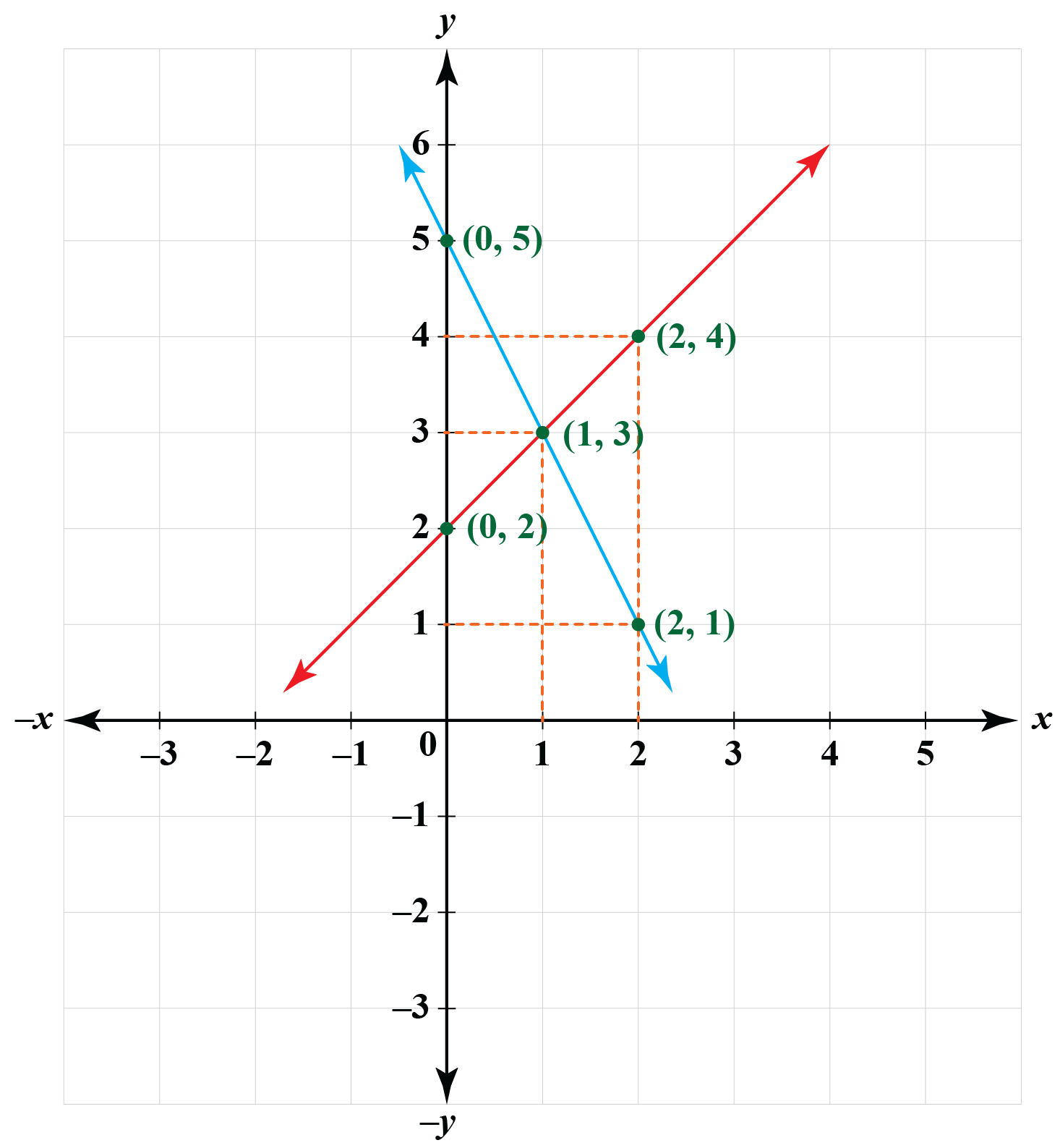The point of intersection is $$A\left( {1,\,\,3} \right)$$, which means that $$x = 1,\;\;y = 3$$ is a solution to the pair of linear equations given by (2). In fact, it is the only solution to the pair, as two non-parallel lines cannot intersect at more than one point.

## How to Solve Linear Equations Using CalculatorTips and Tricks

• You can check directly about the types of solution using the following conditions:
Unique solution (consistent and independent)  $\frac{a_{1}}{a_{2}} \neq \frac{b_{1}}{b_{2}}$
No solution (inconsistent and independent)       $\frac{a_{1}}{a_{2}}=\frac{b_{1}}{b_{2}} \neq \frac{c_{1}}{c_{2}}$

Infinite many solutions (consistent and dependent) $\frac{a_{1}}{a_{2}}=\frac{b_{1}}{b_{2}}=\frac{c_{1}}{c_{2}}$

## Solved Examples

 Example 1

Tom loves to collect ¢2 and ¢5 coins in his piggy bank.He knows that the total sum in his piggy bank is  ¢77, and it has 3 times as many two-cent coins as five-cent coins in it.

He wants to know the exact number of ¢2 and ¢5 coins in his piggy bank.

Can you help him find the count?

Solution

Let the number of $$¢5$$ coins be $$x$$.
The number of $$¢2$$ coins will be $$3x$$.
Total amount = $$5 x + 2 \times 3 x$$
therefore, $5 x + 2 \times 3x = 77$
$5 x + 6x = 77$
$11x = 77$
$x = 7$

 $$\therefore$$ Tom has $$7$$ coins $$¢5$$ of and $$21$$ coins of $$¢2$$
 Example 2

In a river, a boat can travel 40 miles upstream in 2 hours. The same boat can travel 60 miles downstream in 2 hours.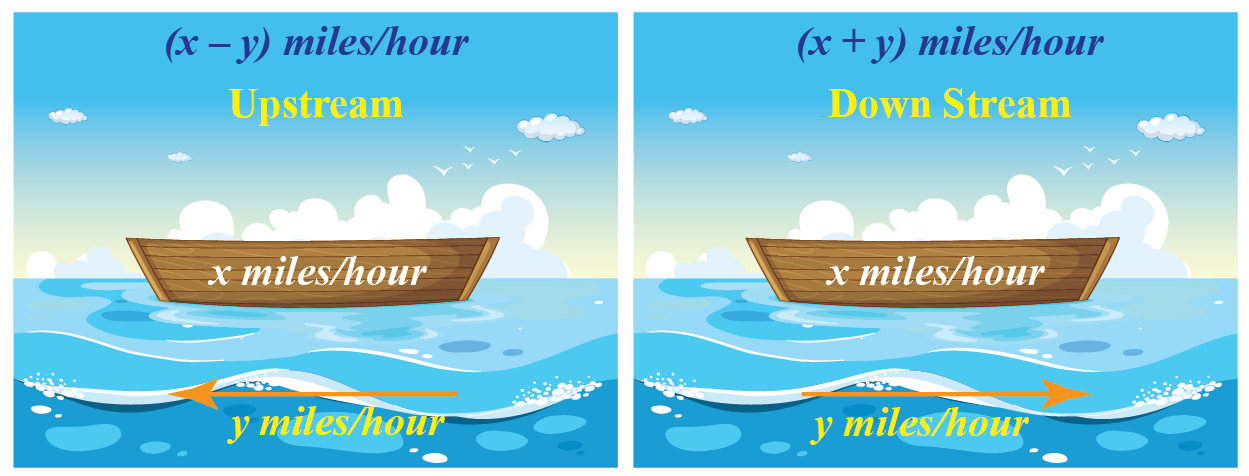• What is the speed of the boat in still water?
• What is the speed of the current?

Solution

Let us assume that:

• the speed of boat in still water = $$x$$ miles per hour
• the speed of current = $$y$$ miles per hour.

During upstream, the current pulls back the boat's speed and the speed of the boat during upstream = $$x-y$$.

During downstream, the current's speed adds to the boat's speed and the speed of the boat during downstream = $$x+y$$.

Thus,

Distance(d) Time(t) Speed Speed=d/t
UPSTREAM 40 miles 2 hr x - y 40/2=20
DOWNSTREAM 60 miles 2 hr x + y 60/2=30

Using the last two columns of the table:

\begin{align} x-y&=20 \,\,\, \rightarrow (1)\\[0.2cm] x+y &=30\,\,\, \rightarrow (2) \end{align}

\begin{align} 2x &= 50\\[0.2cm] x&=25 \end{align}

Substitute this in (2):

\begin{align} y&= 30-25\\[0.2cm] y&=5 \end{align}

 The speed of boat = 25 miles per hour The speed of current = 5 mile per hourChallenging Questions
• Solve the following linear equations:
2 ( x+y) + 3 (x-y) = 13
3 (x+y)  -  2 (x-y ) = 13

## Interactive Questions

Here are a few activities for you to practice. Select/Type your answer and click the "Check Answer" button to see the result.

## Let's Summarize

The mini-lesson targeted the fascinating concept of Solution of a Linear Equations. The math journey around Linear Equations starts with what a student already knows, and goes on to creatively crafting a fresh concept in the young minds. -Done in a way that is not only relatable and easy to grasp but will also stay with them forever. Here lies the magic with Cuemath.

At Cuemath, our team of math experts is dedicated to making learning fun for our favorite readers, the students!

Through an interactive and engaging learning-teaching-learning approach, the teachers explore all angles of a topic.

Be it worksheets, online classes, doubt sessions, or any other form of relation, it’s the logical thinking and smart learning approach that we, at Cuemath, believe in.

## FAQs

### 1. What is the degree of a linear equation?

The degree of a linear equation is 1.

### 2. How many types of linear equations are there?

The three major forms of linear equations are point-slope form, standard form, and intercept form.

### 3. What are linear equations?

A linear equation is an equation in which the variable(s) is(are) with the exponent 1.
Example: $2 x = 23$
$x - y = 5$

### 4. How does one solve the system of linear equations?

We have different methods to solve the system of linear equations:

Graphical method
Substitution method
Cross-multiplication method
Elimination method
Determinants method

### 5. What is Homogeneous linear equations?

In Homogeneous linear equations, all constant terms are zero.

### 6.What is a unique solution?

The unique solution of a system of linear equations is an ordered pair or point which makes equality true in the equation.

### 7. What happens if a pair of linear equations is consistent?

If a pair of linear equations is consistent then the lines either intersect or coincide with (overlap) each other.

Linear Equations
Linear Equations
grade 9 | Questions Set 2
Linear Equations